# Barkhausen Stability Criterion

The  Barkhausen Stability Criterion is simple, intuitive, and wrong. During the study of the phase margin of linear systems, this criterion is often suggested by students grasping for an intuitive understanding of stability. Unfortunately, although counterexamples are easy to provide, I do not know of a satisfying disproof to the Barkhausen Stability Criterion that combats this intuition.

Some textbooks even state the Barkhausen Stability Criterion (although none refer to it by name). In their introduction of the Nyquist Stability Criterion, Chestnut and Meyer state

If in a closed-loop control system with sinusoidal excitation the feedback signal from the controlled variable is in phase and is equal or greater in magnitude to the reference input at any one frequency, the system is unstable.[3, page 142]
They continue to insist that [t]he Nyquist stability criterion presents this fact in a rigorous mathematical form.'' This introduction is completely wrong, and Chestnut and Mayer even provide the Nyquist plot of a perfect counterexample a mere fourteen pages later (while defining conditionally-stable nonlinear systems).

The history of the Barkhausen Stability Criterion is an unfortunate one. In 1921, during his study of feedback oscillators, Barkhausen developed a formula for self-excitation''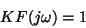where K is an amplifier gain factor andis the frequency dependence of the feedback loop. This equation was originally intended for the determination of the oscillation frequency for use in radio transmitters. However, before conditionally-stable nonlinear systems were understood, it was widely believed that only a single value of K separated stable and unstable regions of behavior. Thus, Barkhausen's Criterion was incorrectly used as a stability criterion, especially in the German literature .

The concept, as stated by Chestnut and Mayer, seems intellectually satisfying. In fact, I've often had students (and professors) ask, But if the gain around the loop is five, and the total phase shift around the loop is exactly zero, then doesn't that imply that any signal around the loop will grow with time?''

This reasoning, although deeply flawed, seems to make sense. There is no shortage of counterexamples, such as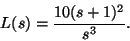Yet quoting a counterexample rarely satisfies the student. Dislodging this intuitive misconception is an uphill battle. Thus, it is worth refuting this argument in every possible way.

## Black's Formula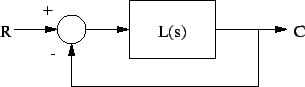Using Black's Formula provides one refutation. For a system with unity negative feedback and loop transfer function L(s), the closed-loop transfer function isClearly, if we know that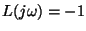at some frequency, then we know that the system is unstable. However, knowing that the loop transfer function is negative and larger than one at one particular frequency gives us no information about stability. For example, if all we know is that, then perhaps L(s)=-5 for all s. Thus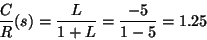Knowing the magnitude and phase at one frequency does not give us enough information to assess stability.

## Evans' Root Locus Method

In a root-locus sense, knowing thatonly means that for less loop gain, we would have closed-loop poles on the imaginary axis. However, we do not know if increasing the gain fivefold moved the closed-loop poles into the left half-plane or into the right half-plane.

## Nyquist Criterion

In a Nyquist sense, knowing thatonly means that there may be encirclements of the s=-1 point. However, this fact alone is not enough information to assess stability. Without more information, we cannot tell how many encirclements there are (or what direction the encirclements are in) or even how many open-loop poles are in the right half-plane. We do not have enough information to determine the number of closed-loop poles in the right half-plane (Z=N+P), because we know neither the number of encirclements N, nor the number of open-loop poles in the right half-plane, P.

## Bottom Line

Unless we know thatfor some frequency, knowing the value of the loop transfer function at one frequency gives us no information about stability. Down with Barkhausen!

Kent H Lundberg
2002-11-14